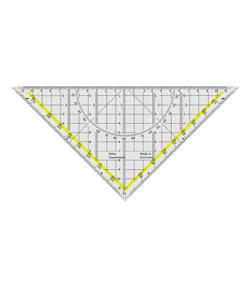Measurement: Mass & Volume

3 Questions | Total Attempts: 9362SettingsA multiple choice quiz related to measurement of mass and volume.

• 1.
A sphere was dropped into water in a graduated cylinder as shown. What is the volume of the sphere?
• A.

15 mL

• B.

25 mL

• C.

40 mL

• D.

65 mL

• 2.
A piece of copper metal is correctly placed on a triple beam balance. The riders are all at the zero mark except for the rider on the 0-10 gram beam which is located at the position shown. What is the mass of the copper metal?
• A.

0.455g

• B.

4.56g

• C.

0.55g

• D.

5.50g

• 3.
The diagram below shows 3 graduated cylinders. The contents of cylinders X and Y are poured into cylinder Z. Which letter represents the amount of liquid that will be in container Z?
• A.

A

• B.

B

• C.

C

• D.

D

Related TopicsBack to top